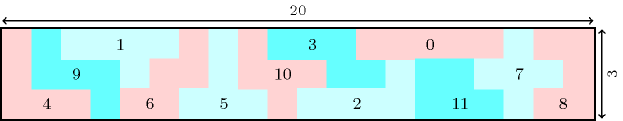### 3.7.184. Pentomino

• $\mathrm{𝚛𝚎𝚐𝚞𝚕𝚊𝚛}$.

A constraint (i.e., $\mathrm{𝚙𝚘𝚕𝚢𝚘𝚖𝚒𝚗𝚘}$) that can be used to model a pentomino. A pentomino is an arrangement of five unit squares that are joined along their edges.

Also denotes a constraint (i.e., $\mathrm{𝚍𝚒𝚏𝚏𝚗}$, $\mathrm{𝚐𝚎𝚘𝚜𝚝}$, $\mathrm{𝚛𝚎𝚐𝚞𝚕𝚊𝚛}$) that can be used for solving tiling problems involving pentominoes. For instance, the $\mathrm{𝚐𝚎𝚘𝚜𝚝}$ and $\mathrm{𝚛𝚎𝚐𝚞𝚕𝚊𝚛}$ constraints where respectively used in  [BeldiceanuCarlssonPoderSadekTruchet07] and in  [LagerkvistPesant08] to solve such tiling problems.

Figure 3.7.50 presents a tiling of a rectangle with distinct pentominoes.

##### Figure 3.7.50. Tiling a rectangle with pentominoes